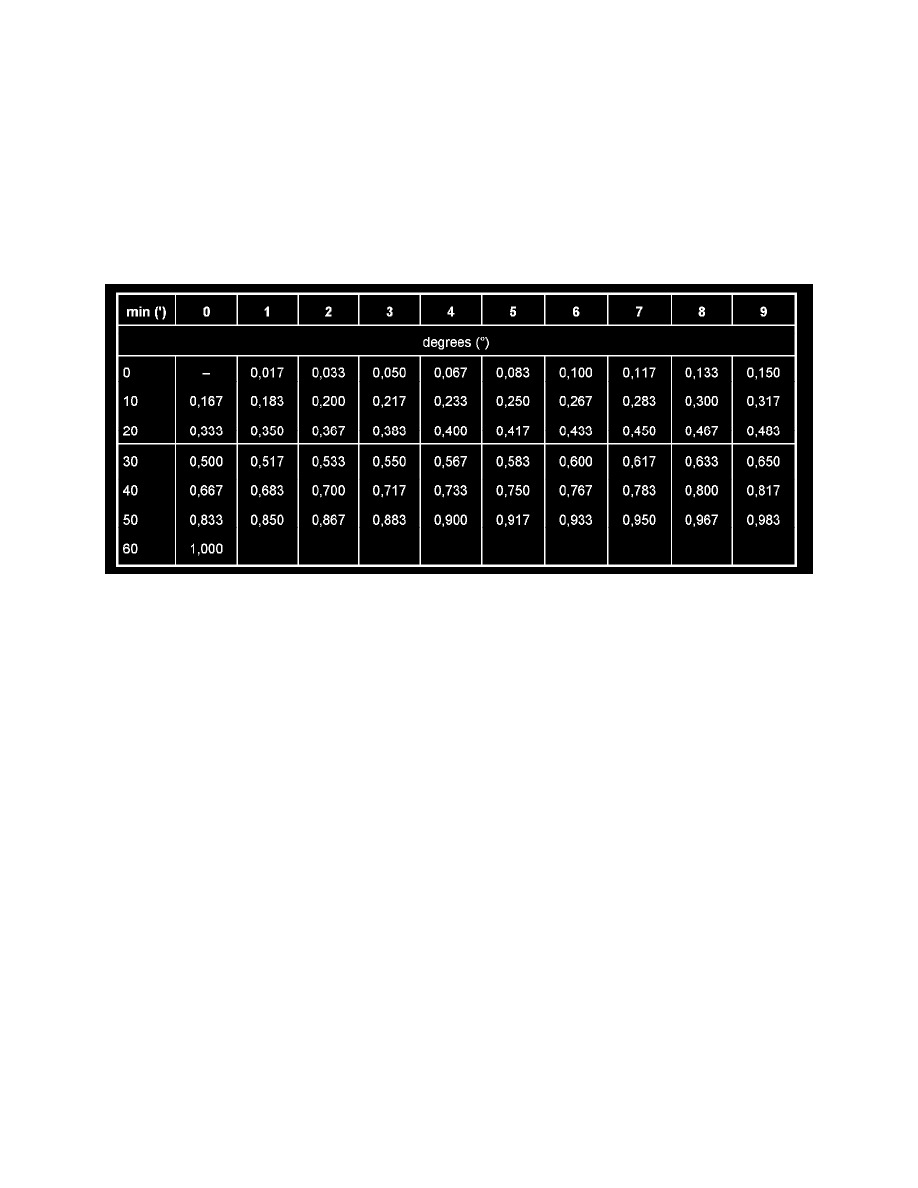# Porsche Workshop Manuals

< PREV PAGE NEXT PAGE >
Page 1Alignment: Specifications
Conversion Table For Wheel Alignment Values

Conversion table for wheel alignment values

Minutes in decimal degrees

^

The wheel alignment values are specified in degrees and minutes (division in 60 units)!

^

In countries in which the decimal degree is widely used, the electronic wheel alignment testers can be converted from degrees/minutes (division in
60 units) to decimal degrees (division in 100 units) by pressing a key (special function). This function is standard for the electronic wheel
alignment testers approved by Porsche!

^

Using the conversion table, minutes (') can be converted into decimal degrees and decimal degrees into minutes (')! To do this, read out the
required values with the help of the coordinates!

Conversion from minutes to decimal degrees (examples)

^

Example 1: toe 17 min (17') = 0.283 decimal degrees according to table. Procedure for this: the imaginary line starting at the top of the 7 minutes
column moving downwards and the line starting on the left in the 10 minutes row moving right meet at point/column 0.283.

^

Example 2: camber 1° 25' (1 degree and 25 min) = 1.417 decimal degrees. This is the result from the table (1 degree or 60 minutes) = 1.000 +
0.417 (25 min).

Conversion from decimal degrees to minutes (examples)

^

Example 1: toe 0.350 decimal degree = 21 min (21'). The decimal value (0.350) is searched for in the table. The coordinates are subsequently
formed from this point/value. Line to the left = 20' and line upwards = 1' (amounts to 20'+ 1').

^

Example 2: camber 1.683 decimal degrees = 1° 41' (1 degree and 41 min). This is attained as follows: divide the value in 1,000 = 1 degree (1°)
and search for the remaining value 0.683 in the table. Then form the coordinates again. Line to the left = 40' and line upwards = 1' (amounts to 40'
+ 1').

### Maintenance > Alignment > System Information > Specifications > Conversion Table For Wheel Alignment Values

< PREV PAGE NEXT PAGE >